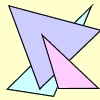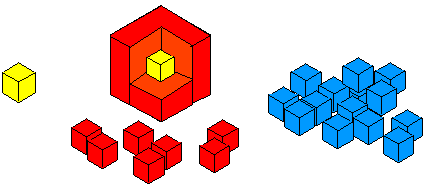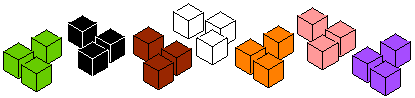#### You may also like### Threesomes

Imagine an infinitely large sheet of square dotty paper on which you can draw triangles of any size you wish (providing each vertex is on a dot). What areas is it/is it not possible to draw?### All in the Mind

Imagine you are suspending a cube from one vertex and allowing it to hang freely. What shape does the surface of the water make around the cube?### Reflecting Squarely

In how many ways can you fit all three pieces together to make shapes with line symmetry?

# Cubes Within Cubes Revisited

##### Age 11 to 14 Challenge Level:
Imagine you have an unlimited supply of interlocking cubes (all the same size) in different colours. Now imagine starting with one yellow cube. This is covered all over with a single layer of red cubes.This is then covered with a layer of blue cubes.

How many red cubes have you used?

How many blue cubes have you used?

Find an easy way of working this out.

Now imagine adding a layer of green cubes.

How many green cubes are needed?

Have you a quick method for working out the amounts that you would need for each layer?Martha thought about the third layer like this:

• The whole of the new cube is a $5$ by $5$ by $5$ cube.
• The inner layers made a $3$ by $3$ by $3$ cube.
• So the new layer is $5^3 - 3^3 = 125 - 27$ cubes.

Can you see it like this?

Using this method, if you have an $x$ by $x$ by $x$ cube, can you explain how to find an expression for the number of cubes needed to make the next layer?

Emma made the third layer like this:

• First she added a $5$ by $5$ plinth to the bottom, and another to the top.
• Then she added a $3$ by $3$ block to each vertical face, and finally four columns of $3$ cubes to the corners.

Can you see it like this?

Using this method, if you have an $x$ by $x$ by $x$ cube, can you explain how to find an expression for the number of cubes needed to make the next layer?

Charlie made the third layer like this:

• First he added a $3$ by $3$ block to the top and another to the bottom.
• Then he made lots of pillars of $5$ cubes, and wrapped $16$ of these round the sides

Can you see it like this?

Using this method, if you have an $x$ by $x$ by $x$ cube, can you explain how to find an expression for the number of cubes needed to make the next layer?

Can you show that all three expressions are equivalent?